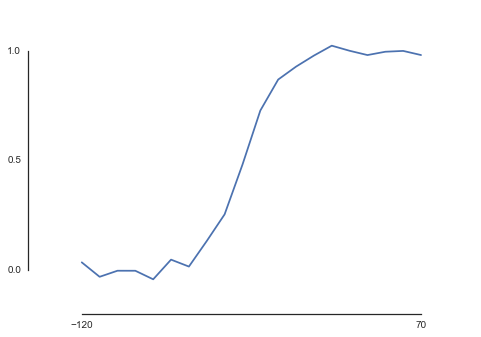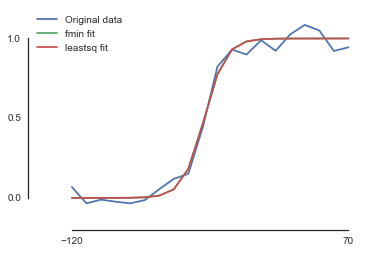# Advanced Curve Fitting

Curve Fitting is a very important feature of science. Here, I describe briefly how to fit a Voltage-Conductance relationship to a single Boltzmann function.
Hi folks, this is my first Jupyter Notebook post. Hopefully, the stuff is rendered on your machine fine. I’m still troubleshooting, however, please feel free to comment.
Starting over with curve fitting, we have to define the function we would like to fit the data to. Here, I am using a sigmoid function, also known as the Boltzmann function. A Boltzmann function is defined as: $$G = \frac{G_{max}}{1 + e^{\frac{V – V_{half}}{k}}}$$. The conductance is abbreviated as $G$, $V_{half}$ is Vhalf; we use here $k$ as Slope.
In :
import numpy as np
from scipy.optimize import minimize, fmin, leastsq
import matplotlib.pyplot as plt
%matplotlib inline
from ipywidgets import interact
import seaborn as sns
sns.set_style('white')

In :
#def fit1exp (x, a, tau, c, y):
#    return a * np.exp (-tau * x + c) + y

def fitBoltzmann (x, Vhalf, slope, Gmax = 1):
return 1 / (1 + np.exp ((x - Vhalf) / slope))

This is a viewer for generating a random data set. Our voltage ranges from -120 to 70 mV in 10 mV steps. We have a conductance between 0 and 1 (i.e. max conductance). Further, we can add some noise.
In :
global x, y

@interact
def bestRandomData (Vhalf:(-120, 70, 10)=-30, Slope:(-20, 20, 1)=-10, Noise:(0., 1., .01)=.5):
global x,y

if Slope:
x = np.linspace(-120, 70, 20)
y = fitBoltzmann(x, Vhalf, Slope)+np.random.randn(*x.shape)*Noise/10
plt.plot(x,y)
plt.ylim([-.2, 1.2])
plt.yticks([0, .5, 1])
plt.xticks([-120, 70])
sns.despine(trim=True)Check the test values
In :
print(x, y)

[-120. -110. -100.  -90.  -80.  -70.  -60.  -50.  -40.  -30.  -20.  -10.
0.   10.   20.   30.   40.   50.   60.   70.] [  1.23394576e-04   3.35350130e-04   9.11051194e-04   2.47262316e-03
6.69285092e-03   1.79862100e-02   4.74258732e-02   1.19202922e-01
2.68941421e-01   5.00000000e-01   7.31058579e-01   8.80797078e-01
9.52574127e-01   9.82013790e-01   9.93307149e-01   9.97527377e-01
9.99088949e-01   9.99664650e-01   9.99876605e-01   9.99954602e-01]

Now our optimize routine…
In :
def fitHelper (p, x, y):
return sum((y-fitBoltzmann(x,*p))**2)

def fitHelperLeastSq (p, x, y):
return fitBoltzmann(x, *p) - y

This is the minimize function. However, it is not suitable for our purpose here. It converges to not reasonable numbers.
In :
methodsSelected = ['Nelder-Mead','Powell','CG','BFGS']

for m in methodsSelected:
print (m)
print ("***********************")
print (minimize(fitHelper, [-30,1], (x, y), method=m))
print ()

Nelder-Mead
***********************
final_simplex: (array([[  854046.55805343,  8583112.78194439],
[  854046.54115917,  8583112.57001939],
[  854046.54906084,  8583112.66913955]]), array([ 3.9876287,  3.9876287,  3.9876287]))
fun: 3.9876287011534379
message: 'Maximum number of function evaluations has been exceeded.'
nfev: 403
nit: 204
status: 1
success: False
x: array([  854046.55805343,  8583112.78194439])

Powell
***********************
direc: array([[  1.00000000e+00,   0.00000000e+00],
[ -1.68950221e-09,   2.07678067e+17]])
fun: 4.0000980266882955
message: 'Optimization terminated successfully.'
nfev: 216
nit: 2
status: 0
success: True
x: array([ -2.74120710e+01,   7.60099730e+17])

CG
***********************
fun: 4.3513320138195333
jac: array([ -5.57303429e-05,  -5.44011593e-04])
message: 'Maximum number of iterations has been exceeded.'
nfev: 23932
nit: 400
njev: 5983
status: 1
success: False
x: array([  37.37931824,  677.26508695])

BFGS
***********************
fun: 17.27991269250084
hess_inv: array([[  1.        ,   0.        ],
[  0.        , -18.46876508]])
jac: array([ 0.        , -0.07494497])
message: 'Desired error not necessarily achieved due to precision loss.'
nfev: 76
nit: 1
njev: 16
status: 2
success: False
x: array([-30.        ,   2.35961914])


Instead, we can use the functions fmin (that is basically the simplex algorithm) and leastsq
In :
fmin_val = fmin(fitHelper, [1, -1], (x,y))

Optimization terminated successfully.
Current function value: 0.163362
Iterations: 66
Function evaluations: 127

In :
leastsq_val = leastsq(fitHelperLeastSq, [-1, -1], (x,y))

And let’s see how they are able to provide use a decent fit
In :
plt.plot(x,y, label='Original data')
plt.plot(x, fitBoltzmann(x, *fmin_val), label='fmin fit')
plt.plot(x, fitBoltzmann(x, *leastsq_val), label='leastsq fit')
plt.legend(loc='best');
plt.xlim([-150, 80])
plt.xticks([-120,70])
plt.yticks([0, 0.5, 1])
sns.despine(trim=True)In :
print("fmin: ", fmin_val)
print("leastsq: ", leastsq_val)

fmin:  [-31.86744084  -6.80339724]
leastsq:  [-31.86741645  -6.80350368]